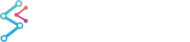﻿ OLD 3D Tutorial 01 - Charting Concepts | JavaScript Chart DocumentationOLD 3D Tutorial 01 - Charting Concepts

## Understanding Charts, Basic Definitions

In order to understand charts, it is necessary to learn some basic concepts first.

Here we will cover:

• Two Dimensional Charts
• 3D charts
• Right Hand Coordinates
• Left-hand Charts
• Camera 3D
• World Coordinates

## Two Dimensional Charts

The Cartesian Plane is how data is represented in two dimensions.

The two dimensions are height and width. In mathematics these are the x and y axes, respectively. A 3D chart adds depth, which is labelled Z. The cartesian plane looks this this, where the pink line is the function y=f(x), where y is some function, like sales = 2 * ad budget. The points x-y are given (x,y). The origin is the point where x=0 and y=0.## 3D Charts

In 3D charts, we introduce a 3rd axis, z. Points on the chart are (x,y,z). This z axis is at a right angle to x and y. This creates the illusion that the graph is 3 dimensional.

## Right-Hand Coordinates

When the z axis points outward this is called a right-handed coordinate system. When it points inward it is called left-handed.

In the right-handed coordinate system positive rotation about the axis is counter clockwise.Charts in SciChart are left-hand by default with the y axis point up, the x axis horizontal, and the z axis pointing inward. Rotation is clockwise. Rotation about the vertical axis is called yaw and rotation about the horizontal axis is called pitch, just like the terms used in aviation.

## Left-Hand Coordinates

In the chart below the chart is rotated (yaw) 45 degrees in the clockwise direction. In the chart on the right it is 90 degrees. For both charts the rotation about the axis is 45 degrees, which is the default value.

45 degrees

90 degreesHere is the xyz origin and axis in the middle of the box as it is when using the World Coordinate System## Camera 3D

The Camera is a SciChart object. Think of this as the location from which you are viewing the chart, just like you were floating above the surface and looking down at it with a pair of binoculars. We explain this using the concepts below. A camera has an xyz position and pitch (up and down) and yaw (rotation).

Camera coordinates are three dimensional world coordinates projected on a two dimensional plane.This graphic shows how you can change the pitch and yaw of the camera. Notice that the red, green, and blue xyz graphic to show the orientation of the world coordinate system.## World Coordinates

World coordinates are the (x,y,z) coordinates used to locate an object in 3D space. You can transform camera coordinates to world coordinates and vice versa. SciChart does this mathematics when it renders a 3D object onto 2D surface of a tablet or mobile device screen.

In terms of charting, the origin (0,0,0) is located in the middle of the box shown below which represents 3D space.